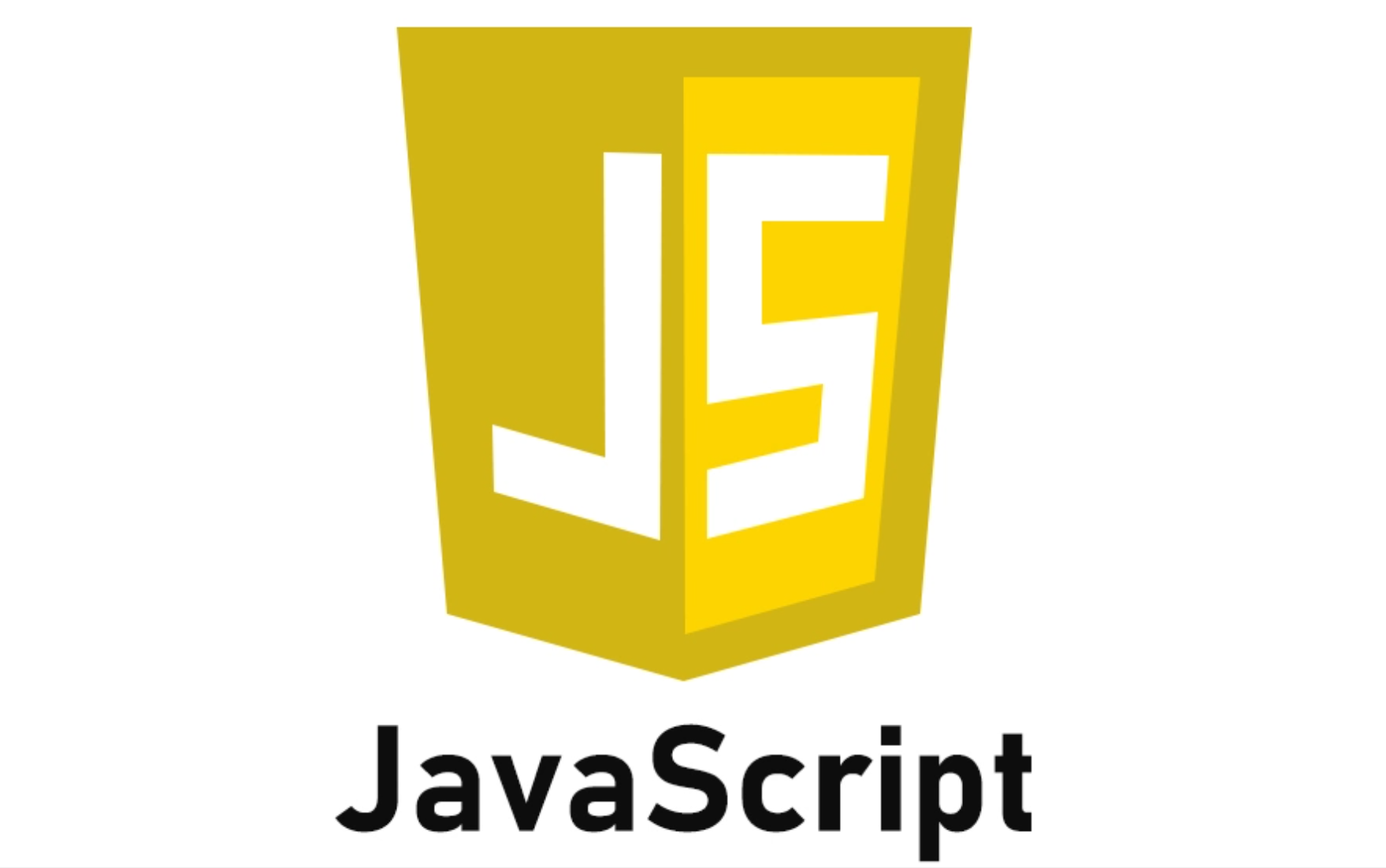# 【JavaScript】：有关 js 类型转换的那些事...

• 2022 年 8 月 25 日
广东
• 本文字数：1291 字

阅读完需：约 4 分钟• 简单类型转换

# 内容

JavaScript 的类型转换灵活多样，无论是隐式类型转换还是显示类型转换，方法都有很多。具体请往下看：

## 数字转换为字符串

JavaScript 中，如果某处期望使用数字，则会将字符串转换为数字。

"PI:" + 3.14  // => "PI:3.14"

var data = 19;bStr = data.toString(2);      // => "10011"oStr = "0" + data.toString(8);      // => "023"hStr = "0x" + data.toString(16);    // => "0x13"

• toExponential(n) ：使用指数计数法，此时小数点前只有一位，小数点后有几位由参数 n 决定

var data = 967.12345;data.toFixed(0)  // => "967"data.toFixed(3)  // => "967.123"data.toFixed(6)  // => "967.123450"

• toFixed(n) ：控制输出数值小数点后的位数，由参数 n 决定

var data = 967.12345;data.toExponential(0);  // => "1e+3"data.toExponential(3);  // => "9.671e+2"data.toExponential(6);  // => "9.671235e+2"

## 字符串转换为数字

"4" * "6"  // => 24"5" / "2"  // => 2.5"15" - "5" // => 10

"value" * "5"  // => NaN

parseInt("110")         // => 110parseInt("010")         // => 10parseInt("30而立")         // => 30parseInt("0x152")        // => 338parseInt("1111", 2)     // => 15parseInt("a", 16)       // => 10parseInt("2.22")       // => 2parseInt("0.18")       // => 0parseFloat("0.18")       // => 0.18parseFloat("#3.14")        // => NaNparseFloat(" 6.66的红包")   // => 6.66

## 转换为布尔值

JavaScript 中，会根据需求自行转换为布尔值。比如将一些真值转换为 true，将一些假值转换为 false。举例：能转换为false的有空字符串""，关键字nullundefined，数字0（和-0）。

# 写在最后@珠海科技学院

## 评论